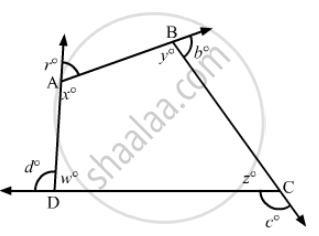Advertisement Remove all ads

# The Sides of a Quadrilateral Are Produced in Order. What is the Sum of the Four Exterior Angles? - Mathematics

Short Note

The sides of a quadrilateral are produced in order. What is the sum of the four exterior angles?

Advertisement Remove all ads

#### Solution

$\text{ The sides of the quadrilateral ABCD are produced in order (according to figure) . }$

$\text{ Now, we need to find the sum of the exterior angles } .$

$\text{ Since the angles made on the same side of straight line are } 180 °, \text{ i . e . , linear pair, we have: }$

$a + x + b + y + c + z + w + d = 180° + 180° + 180° + 180°= 720°$

$OR$

$\text{ Sum of the interior angles + sum of exterior the angles } = 180 ° \times 4 = 720°$

$\text{ Since the sum of the interior angles of a quadrilateral is } 360° , \text{ we have } :$

$w + x + y + z = 360°$

$\text{ Substituing the value, we get } :$

$a + b + c + d = 360°$

$\therefore \text{ Sum of the exterior angles } = 360 °$Is there an error in this question or solution?
Advertisement Remove all ads

#### APPEARS IN

RD Sharma Class 8 Maths
Chapter 16 Understanding Shapes-II (Quadrilaterals)
Exercise 16.1 | Q 14 | Page 16
Advertisement Remove all ads
Advertisement Remove all ads
Share
Notifications

View all notifications

Forgot password?
Course